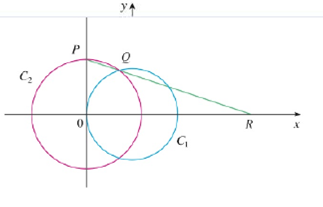Chapter 2.3, Problem 66E

Chapter
Section
Textbook Problem

The figure shows a fixed circle C1, with equation (x – 1)2 + y2 = 1 and a shrinking circle c, with radius r and center the origin. P is the point (0, r ), Q is the upper point of intersection of the two circles, and R is the point of intersection of the line PQ and the x-axis. What happens to R as C2 shrinks, that is, as r →0+?To determine

To find: The point R when the circle C2 shrinks (as r0+).

Explanation

Given:

The equation of the fixed circle C1 is (x1)2+y2=1.

The equation of the shrinking circle C2, x2+y2=r2.

P be the point (0,r), Q be the upper point of intersection of the two circles and R be the point of intersection of the line PQ and the x axis.

Formula used:

Slope of the line: m=y2y1x2x1

Equation of line between two points: (yy1)=m(xx1)

Difference of square formula: (a2b2)=(a+b)(ab)

Limit Laws:

Suppose that c is a constant and the limits limxaf(x) and limxag(x) exist. Then,

Limit law 1: limxa[f(x)+g(x)]=limxaf(x)+limxag(x)

Limit law 2: limxa[f(x)g(x)]=limxaf(x)limxag(x)

Limit law 3: limxa[cf(x)]=climxaf(x)

Limit law 7: limxac=c

Limit law 9: limxaxn=an where n is a positive integer.

Limit law 11: limxaf(x)n=limxaf(x)n where n is a positive integer, if n is even, assume that limxaf(x)>0.

Calculation:

Given that the point Q is the point of intersection of the circles C1 and C2.

(x1)2+y2=1 (1)

x2+y2=r2 (2)

Eliminate y from equation (1) and (2),

(x1)2+y2(x2+y2)=1r2(x1)2x2=1r2x22x+1x2=1r22x+1=1r22x=r2

Isolate, x.

2x=r2x=r22

Substitute x=r22 in equation (2),

(r22)2+y2=r2r44+y2=r2y2=r2r44y2=r2(1r24)

Take square root on both sides,

y=r1r24

Therefore, the point Q is (12r2,r114r2).

Thus, the coordinates of P(x1,y1) and Q(x2,y2) are (0,r) and (12r2,r114r2).

Obtain the slope of the line passing through the points P and Q by using the slope of the line formula.

m=r114r2r12r20=r(114r21)12r2=2(114r21)r

Obtain the equation of the line joining the points P and Q by using the equation of line between two points as follows.

(yy1)=m(xx1)yr=2(114r21)r(x0)

yr=2(114r21)rx (3)

Note that, R is the point of intersection of the line PQ and the x axis

Still sussing out bartleby?

Check out a sample textbook solution.

See a sample solution

The Solution to Your Study Problems

Bartleby provides explanations to thousands of textbook problems written by our experts, many with advanced degrees!

Get Started

Use the definition of derivative to prove that limx0ln(1+x)x=1

Single Variable Calculus: Early Transcendentals, Volume I

Find an equation of the vertical line that passes through (0, 5).

Applied Calculus for the Managerial, Life, and Social Sciences: A Brief Approach

Write 28,500 in scientific notation.

Elementary Technical Mathematics

In Problems 25-30, suppose that 25. What is ?

Mathematical Applications for the Management, Life, and Social Sciences

The third partial sum of is:

Study Guide for Stewart's Multivariable Calculus, 8th